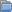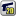﻿ Unreal Software - Thread: New Problm

# ForumTrashNew Problm

#New Problm

Goto Page1ubmasterr
BANNED
OfflineHi guys i have new problem.

Code:
Code:
1
2
3
4
5
6
7
8
9
10
function BLOCKattack(id)
for _, b in ipairs(BLOCKS) do
local x, y = player(id, 'x'), player(id, 'y')
local dist = math.sqrt((b.x-x)^2+(b.y-y)^2)
if dist <= 32 then
Block:damage(id, math.ceil(20*((PLAYERS[id].tmp.ppower)/math.random(60, 140))))
end
end
end

I havent lua error but not working. why?/where are problem?.

thx all guys.

Trashed because of bad title (and you know that it's bad, you wrote problm instead of problem to use this bad title anyway) /DC
Dimas541
User
Offlineubmasterr has written:
Hi guys i have new problem.

Code:
Code:
1
2
3
4
5
6
7
8
9
10
function BLOCKattack(id)
for _, b in ipairs(BLOCKS) do
local x, y = player(id, 'x'), player(id, 'y')
local dist = math.sqrt((b.x-x)^2+(b.y-y)^2)
if dist <= 32 then
Block:damage(id, math.ceil(20*((PLAYERS[id].tmp.ppower)/math.random(60, 140))))
end
end
end

I havent lua error but not working. why?/where are problem?.

thx all guys.

tibia code block system -.-File does not exist (14217) <-- My Tibia EditedCarMods (20) <-- My CarMods1﻿